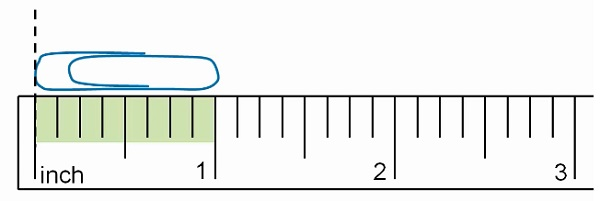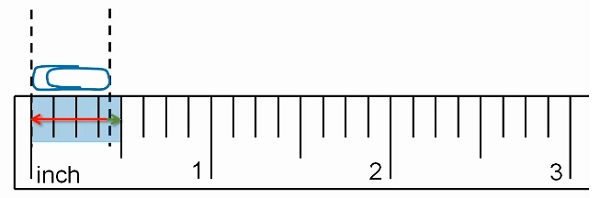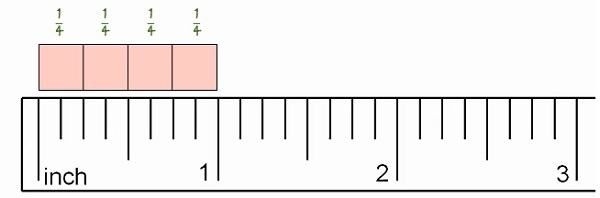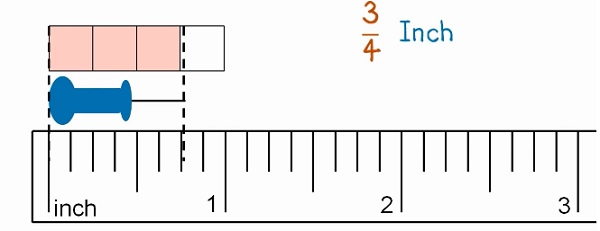# Measuring Length to the Nearest Quarter or Half Inch

Measuring length to the nearest half inch

This paper clip is slightly more than one-inch longWe see that this paper clip is closer to the one-inch mark than one and half inch mark. So the length to the nearest half inch is one inch only.

This paper clip is closer to the half inch mark than the zero-inch mark. So its length to the nearest half inch is 0.5 inch only.Measuring length to the nearest quarter inchWhile measuring to the nearest quarter inch, we think of an inch as being divided into four equal parts each equal to a $\frac{1}{4}^{th}$ of an inch.The length of this push pin is closer to 3 quarters than to 4 quarters of an inch. Hence its length to nearest quarter of an inch is three quarters of an inch or $\frac{3}{4}$-inch.

Measure the length of the object to the nearest half inch.### Solution

Step 1:

The reading is nearer to $2\frac{1}{2}$ mark than to 3 mark.

Step 2:

So, the length of the object to nearest half inch is $2\frac{1}{2}$ inches.

Measure the length of the object to the nearest quarter inch.### Solution

Step 1:

The reading is nearer to $3\frac{3}{4}$ mark than to $3\frac{2}{4}$ mark.

Step 2:

So, the length of the object to nearest quarter inch is $3\frac{3}{4}$ inches.